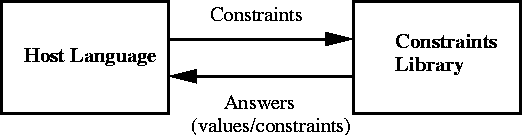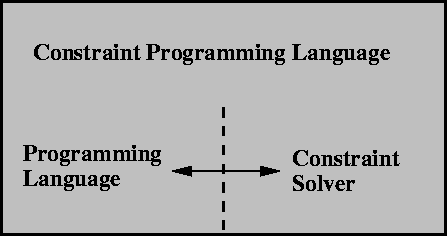Next: An Example: SEND + Up: What is Constraint (Logic) Previous: Why Constraints and Programming?

# Constraint-Programming Language Interfaces

There are two basic ways of using constraints from inside a programming language. One is providing a library with data structures and classes which implements objects such as variables, equations, etc., and methods to combine formulae using mathematical (or other) operations to give more formulae, combining formulae using mathematical relations to give equations, putting together equations in sets, testing their solvability (and trying to solve them), etc. This is exemplified in Figure 1.1.As good as it can be, it will not integrate seamlessly with the semantics of the host language, for the constrained variables are not language variables, and the same happens with the equations, relationships: the do not belong to the language. For that, an alternative path to coupling constraints and programming is making the language semantics richer by adding high-level mathematical properties to the basic building blocks of the language: variables can now be related to other variables, and can hold non-definite values. Constraint solving is performed automatically as program execution progresses, since the constraint solver is part of the runtime system. This is depicted in Figure 1.2.It is not surprising that functional and logic languages (specially the latter ones, because they already provide logical variables and implicit search) are the ones more amenable to this approach: their mathematical foundation and independence from the machine offer leeway to for adapting their semantics self-congruently.

Regardless of the approach taken towards the construction of a constraint language, there are some essential services that such a language must provide:

• A solver, which solves equations or communicates their non-solvability (the way this is done depends on the actual interface with the host language).
• Means to express constraints, formulas, etc. from the language.
• An interface to the solver, which allows constraints to be passed to it, and, upon successful constraint solving, asking for the values assigned to the constraint variables.Next: An Example: SEND + Up: What is Constraint (Logic) Previous: Why Constraints and Programming?
MCL
1998-12-03